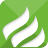# 关于js中关联数组的使用问题

2012-10-26 12:33:31 | 浏览量：755

function test1() {
var array1 = { "A":"a","B":"b","C":"c" };
for (key in array1) {  // 能够正常输出数组
alert('=1=1=====' + key);
alert('=1=2=====' + array1[key]);
}
}
function test2() {
var array2 = new Array();
array2["A"] = "a";
array2["B"] = "b";
array2["C"] = "c";
for (key in array2) {   // 在输出正确数组前，会先输出许多函数信息
alert('==1=====' + key);
alert('==2=====' + array2[key]);
}
}

array2["A"]="a"其实就是array2.A="a";

array2.A="a"
array2.B="b"
array2.C="c"

var array2 ＝ {};或var array2 = new Object();  的方式！

0.3662s